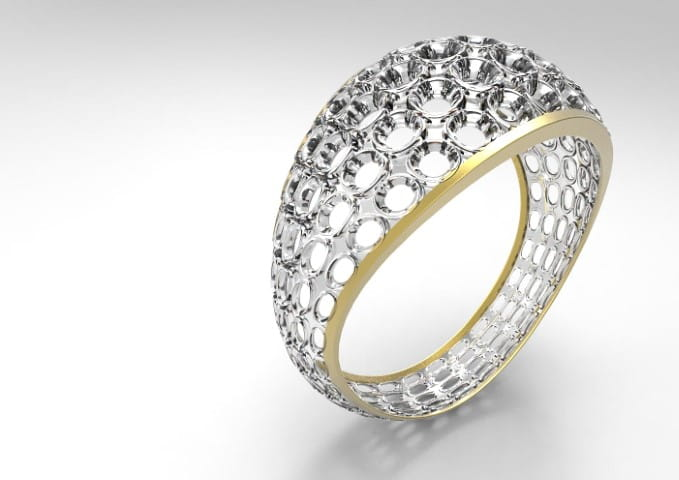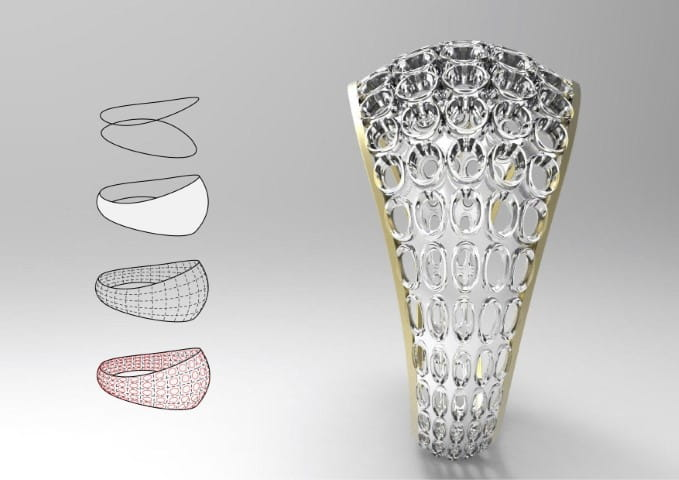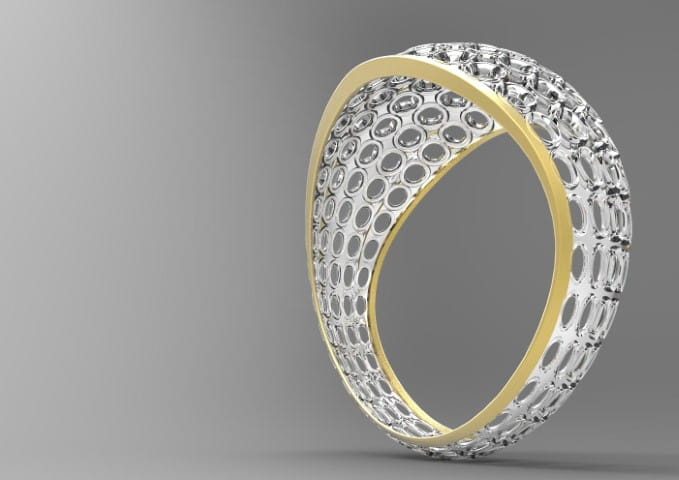# A Ring

back
Computational design of a ring.
Chen, 2016-01-16

This is an assignment of computational design course. A sketchy design of a ring.

Basically I played with surface domain: sub-divide the surface and create circles on each surface domain. Size and thickness of the circle changes according to the divided surface.code in rhinoscript:

``````import rhinoscriptsyntax as rs

rs.EnableRedraw(False)
#Creat Rail 1
Crv1Pts=[(-40,0,6),(0,40,3),(40,0,20),(0,-40,3),(-40,0,6)]
#Creat Rail 2
Crv2Pts=[(-40,0,-6),(0,40,-3),(40,0,-20),(0,-40,-3),(-40,0,-6)]
#Creat Rings
#Creat profile
StartPt1 = rs.CurveStartPoint(Crv1)
StartPt2 = rs.CurveStartPoint(Crv2)
#Creat Surface
rs.RebuildSurface(strSrf,(3,3),(30,8))
#Get Surface Domain
DomainU = rs.SurfaceDomain(strSrf,0)
DomainV = rs.SurfaceDomain(strSrf,1)
#get circles on each unit of surface
arrCir = []
arrPt = []
for i in range(1,int(DomainU)+1):
for j in range(1,int(DomainV)+1):
pt1 = rs.EvaluateSurface(strSrf,i-1,j-1)
pt2 = rs.EvaluateSurface(strSrf,i-1,j)
pt3 = rs.EvaluateSurface(strSrf,i,j)
pt4 = rs.EvaluateSurface(strSrf,i,j-1)
ptcenter = (pt1+pt2+pt3+pt4)/4
crv = rs.ScaleObject(crv,ptcenter,[0.7,0.7,0.7])
pullcrv = rs.PullCurve(strSrf,crv,True)
if len(pullcrv)>1:
joinedcrv = rs.JoinCurves(pullcrv,True)
else:
joinedcrv = pullcrv
arrCir.append(joinedcrv)
arrPt.append(ptcenter)

#get length range of circles
arrLength = [rs.CurveLength(i) for i in arrCir]
SortLength = arrLength[:]
SortLength.sort()
Lenmin = SortLength
Lenmax = SortLength[-1]

#define a mapping tool
def translate(OldValue, OldMin, OldMax, NewMin, NewMax):
OldRange = (OldMax - OldMin)
if (OldRange == 0):
NewValue = NewMin
else:
NewRange = (NewMax - NewMin)
NewValue = (((OldValue - OldMin) * NewRange) / OldRange) + NewMin
return NewValue

for i in range(len(arrCir)):
#move circle through normal
PtUV = rs.SurfaceClosestPoint(strSrf,arrPt[i])
Pt = rs.EvaluateSurface(strSrf,PtUV,PtUV)
Normal = rs.SurfaceNormal(strSrf,PtUV)
UnitNormal = rs.VectorUnitize(Normal)
Distance = translate(arrLength[i],Lenmin,Lenmax,0.5,3)
Vector = rs.VectorScale(UnitNormal,Distance)
#scale circle accordingly
scale = translate(arrLength[i],Lenmin,Lenmax,1.3,1.6)
cir = rs.ScaleObject(arrCir[i],Pt,[scale,scale,scale],True)
ForwardCircle = rs.CopyObject(cir,Vector)
# vec to back side
Vec = rs.VectorScale(UnitNormal,0.3)
ReversedVec = rs.VectorReverse(Vec)
BackCircle = rs.MoveObject(cir,ReversedVec)